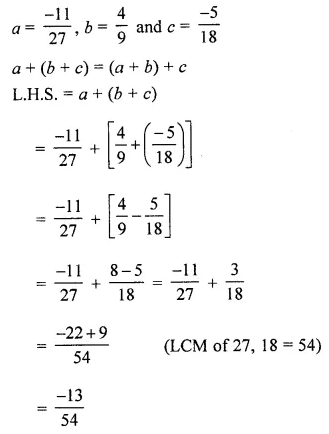## ML Aggarwal Class 8 Solutions for ICSE Maths Chapter 1 Rational Numbers Ex 1.1

Question 1.Solution: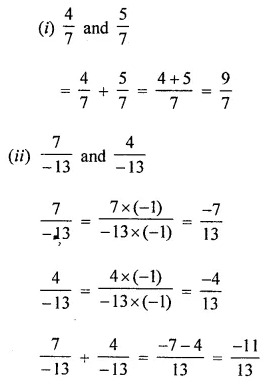Question 2.
Simplify: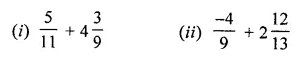Solution: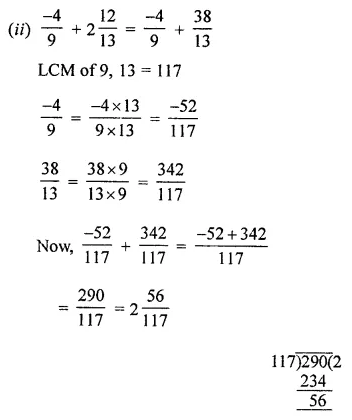Question 3.
Verify commutative property of addition for the following pairs of rational numbers:Solution: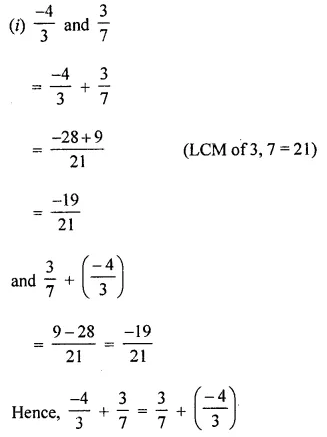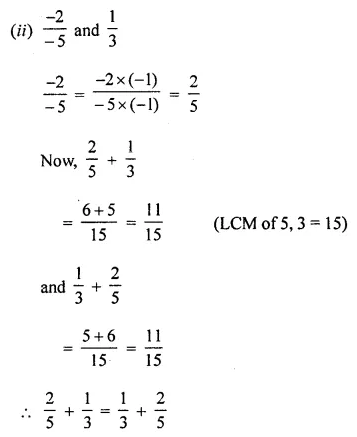Question 4.
Find the additive inverse of the following rational numbers:
(i) $$\frac { 2 }{ -3 }$$
(ii) $$\frac { -7 }{ -12 }$$
Solution: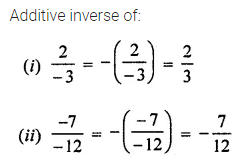Question 5.
Verify that -(-x) = x for
(i) x = $$\frac { 10 }{ 13 }$$
(ii) x = $$\frac { -15 }{ 17 }$$
Solution: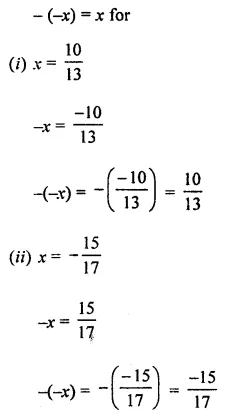Question 6.
Using appropriate properties of addition, find the following: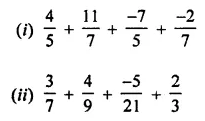Solution: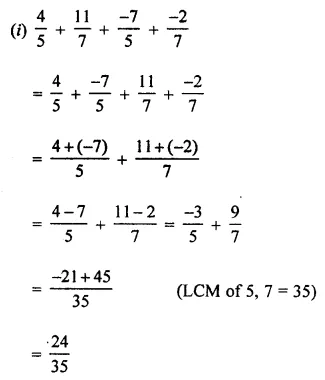Question 7.
Fill in the following blanks:Solution:Question 8.
If a = $$\frac { -11 }{ 27 }$$, b = $$\frac { 4 }{ 9 }$$ and c = $$\frac { -5 }{ 18 }$$, then verify that a + (b + c) = (a + b) + c.
Solution: